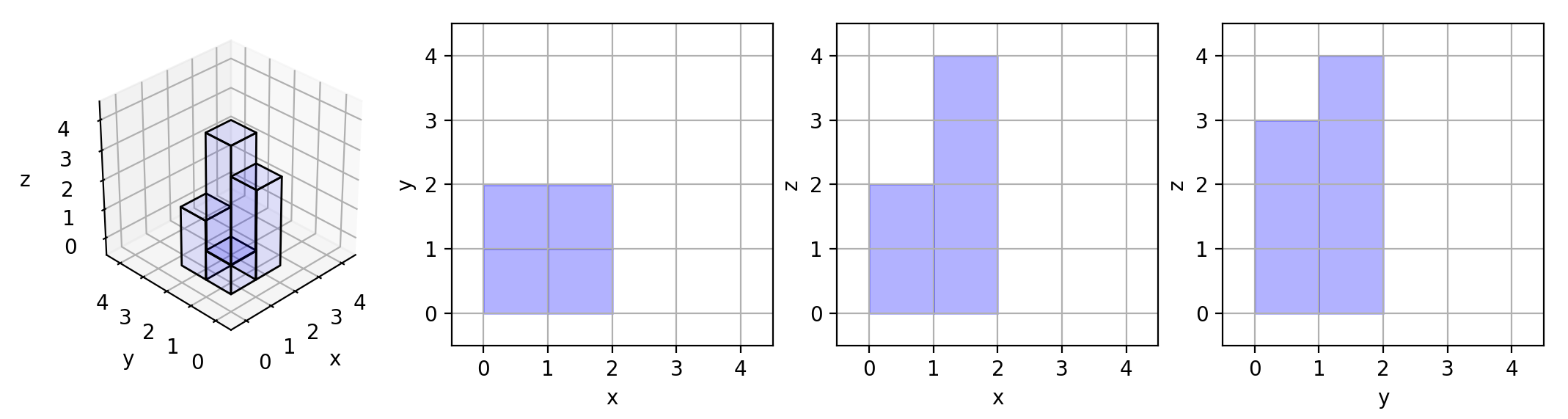883. Projection Area of 3D Shapes

You are given an `n x n` `grid` where we place some `1 x 1 x 1` cubes that are axis-aligned with the `x`, `y`, and `z` axes.

Each value `v = grid[i][j]` represents a tower of `v` cubes placed on top of the cell `(i, j)`.

We view the projection of these cubes onto the `xy`, `yz`, and `zx` planes.

A projection is like a shadow, that maps our 3-dimensional figure to a 2-dimensional plane. We are viewing the "shadow" when looking at the cubes from the top, the front, and the side.

Return the total area of all three projections.

Example 1:```Input: grid = [[1,2],[3,4]]
Output: 17
Explanation: Here are the three projections ("shadows") of the shape made with each axis-aligned plane.
```

Example 2:

```Input: grid = []
Output: 5
```

Example 3:

```Input: grid = [[1,0],[0,2]]
Output: 8
```

Example 4:

```Input: grid = [[1,1,1],[1,0,1],[1,1,1]]
Output: 14
```

Example 5:

```Input: grid = [[2,2,2],[2,1,2],[2,2,2]]
Output: 21
```

Constraints:

• `n == grid.length`
• `n == grid[i].length`
• `1 <= n <= 50`
• `0 <= grid[i][j] <= 50`

883. Projection Area of 3D Shapes
``````struct Solution;

impl Solution {
fn projection_area(grid: Vec<Vec<i32>>) -> i32 {
let mut sum_z: i32 = 0;
let n = grid.len();
let m = grid.len();
let mut x = vec![0; n];
let mut y = vec![0; m];
for i in 0..n {
for j in 0..m {
if grid[i][j] != 0 {
sum_z += 1;
}
x[i] = i32::max(x[i], grid[i][j]);
y[j] = i32::max(y[j], grid[i][j]);
}
}

let sum_x: i32 = x.iter().sum();
let sum_y: i32 = y.iter().sum();
sum_x + sum_y + sum_z
}
}

#[test]
fn test() {
let grid: Vec<Vec<i32>> = vec_vec_i32![];
let res = 5;
assert_eq!(Solution::projection_area(grid), res);
let grid: Vec<Vec<i32>> = vec_vec_i32![[1, 2], [3, 4]];
let res = 17;
assert_eq!(Solution::projection_area(grid), res);
let grid: Vec<Vec<i32>> = vec_vec_i32![[1, 0], [0, 2]];
let res = 8;
assert_eq!(Solution::projection_area(grid), res);
let grid: Vec<Vec<i32>> = vec_vec_i32![[1, 1, 1], [1, 0, 1], [1, 1, 1]];
let res = 14;
assert_eq!(Solution::projection_area(grid), res);
let grid: Vec<Vec<i32>> = vec_vec_i32![[2, 2, 2], [2, 1, 2], [2, 2, 2]];
let res = 21;
assert_eq!(Solution::projection_area(grid), res);
}
``````